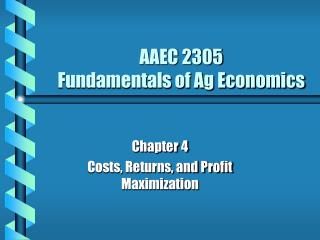DownloadDownload PresentationAAEC 2305 Fundamentals of Ag Economics

# AAEC 2305 Fundamentals of Ag Economics

Download Presentation## AAEC 2305 Fundamentals of Ag Economics

- - - - - - - - - - - - - - - - - - - - - - - - - - - E N D - - - - - - - - - - - - - - - - - - - - - - - - - - -
##### Presentation Transcript

1. AAEC 2305Fundamentals of Ag Economics Chapter 4 Costs, Returns, and Profit Maximization

2. Introduction • A manager’s goal is to determine how much to produce in order to maximize profits. • In Chapter 3, we established Stage II is the rational stage of pdn, but price information (cost) is necessary to determine at which point in Stage II to produce. • Profit is affected not only by how much is produced, but also by the costs of generating that pdn.

3. Objective • Objective of Chapter 4 is to introduce cost and revenue relationships into production to evaluate profit maximization. • Combine what we know about the physical pdn process with input price information to examine relationship between costs of production and level of output produced.

4. Assumptions • 1) Firms seek to maximize π • 2) One product, one pdn method • 3) One variable input, all others are fixed or held constant • 4) Perfect Information • 5) Price taker

5. Cost Definitions • Costs of Pdn or Economic Costs: The payments that a firm must make to attract inputs and keep them from being used to produce other products. • Explicit Costs - Normal out of pocket costs of inputs used in pdn • Implicit Costs- Costs associated with inputs owned by the firm (i.e., opportunity costs - ex., land)

6. Fixed vs. Variable Costs • Fixed Costs: Costs which do not vary with the level of pdn - These costs are associated with the fixed factors of pdn. • Incurred regardless whether any output is produced • Variable Costs: Costs that vary as the output level changes - These costs are associated with variable factors of pdn.

7. Cost Relationships in Pdn • Costs Based on Total Output • 1) Total Fixed Costs (TFC) • 2) Total Variable Costs (TVC) • 3) Total Costs (TC) • TFC (overhead costs) - costs of inputs (implicit & explicit) that are fixed in the SR & do not change as the output level changes.

8. Cost Relationships in Pdn • TVC - costs of inputs (implicit & explicit) that are variable in the SR, and change as output level changes. • Calculated by summing the cost of each variable input used • TVC = (PX1X1) + (PX2X2) + . . . . + (PXnXn) • For One Variable Input: • TVC = PXX

9. Cost Relationships in Pdn • TC - sum of TFC & TVC • TC = TFC + TVC

10. Total Cost Curves(Assume TFC = \$10 and Px = 4 X Y TFC TVC TC

11. Cost Relationships in Pdn • Costs Based on Per-Unit Output • 1) Average Fixed Costs (AFC) • 2) Average Variable Costs (AVC) • 3) Average Total Costs (ATC) • AFC - Average cost of fixed inputs per unit of output • AFC = TFC / Y

12. Cost Relationships in Pdn • AVC - Average cost of variable inputs per unit of output • AVC = TVC / Y • ATC - Average total cost per unit of output • ATC = TC / Y

13. Cost Relationships in Pdn • MC - Increase in total cost necessary to produce one more unit of output • MC = ΔTC / ΔY = ΔTVC / ΔY

14. Cost Curves(Assume TFC = \$10 and Px = 4 X Y TFC TVC TC AFC AVC ATC MC

15. Summary of Relationships Between AFC, AVC, ATC, & MC Curves • AFC is a continuously decreasing function w/ the shape of a rectangular hyperbola • AVC & ATC curves are U-shaped (representing increasing & decreasing returns) • The vertical distance between ATC & AVC at each output level is equal to AFC

16. Summary of Relationships Between AFC, AVC, ATC, & MC Curves • MC crosses both AVC & ATC from below at their respective minimums • ATC is also referred to as Average Cost

17. Cost Curves & Pdn Process • The cost curves are derived directly from the pdn process. • Therefore, the pdn function can be transferred directly to the cost curves • APP & AVC and MPP & MC are mirror images of each other

18. Summary of Relationships • When MPP > APP (APP is increasing)  • MC < AVC (AVC is decreasing) • When MPP = APP (APP is max)  MC = AVC (AVC is min) • When MPP < APP (APP is decreasing)  • MC > AVC (AVC is increasing)

19. Mathematical Relationships • MC = ΔTC / Δ Y = PX / MPP • AVC = TVC / Y = PX / APP

20. Changes in Input Price • Input Price Increase • The cost of producing each output level increases - TVC & TC shift upward & left; TFC remains unchanged - AVC, AC, & MC shift upward & left • Input Price Decrease (or technological innovation increases productivity) • The cost of producing same amount of output decreases - TVC & TC shift downward & right - AVC, ATC, & MC shift downward & right